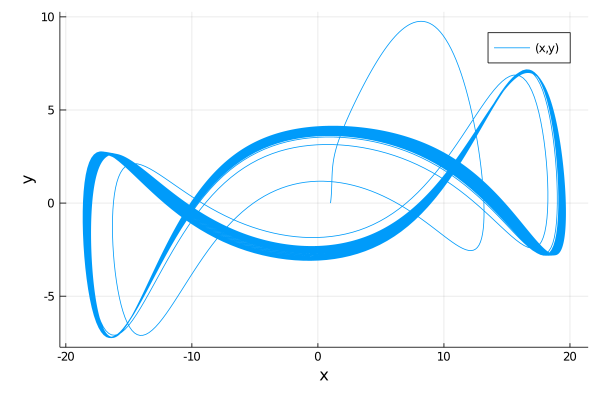# Automatic Transformation of Nth Order ODEs to 1st Order ODEs

ModelingToolkit has a system for transformations of mathematical systems. These transformations allow for symbolically changing the representation of the model to problems that are easier to numerically solve. One simple to demonstrate transformation is the ode_order_lowering transformation that sends an Nth order ODE to a 1st order ODE.

To see this, let's define a second order riff on the Lorenz equations. We utilize the derivative operator twice here to define the second order:

using ModelingToolkit, OrdinaryDiffEq

@parameters σ ρ β
@variables t x(t) y(t) z(t)
D = Differential(t)

eqs = [D(D(x)) ~ σ*(y-x),
D(y) ~ x*(ρ-z)-y,
D(z) ~ x*y - β*z]

@named sys = ODESystem(eqs)

Note that we could've used an alternative syntax for 2nd order, i.e. D = Differential(t)^2 and then E(x) would be the second derivative, and this syntax extends to N-th order. Also, we can use * or ∘ to compose Differentials, like Differential(t) * Differential(x).

Now let's transform this into the ODESystem of first order components. We do this by simply calling ode_order_lowering:

sys = ode_order_lowering(sys)

Now we can directly numerically solve the lowered system. Note that, following the original problem, the solution requires knowing the initial condition for x', and thus we include that in our input specification:

u0 = [D(x) => 2.0,
x => 1.0,
y => 0.0,
z => 0.0]

p  = [σ => 28.0,
ρ => 10.0,
β => 8/3]

tspan = (0.0,100.0)
prob = ODEProblem(sys,u0,tspan,p,jac=true)
sol = solve(prob,Tsit5())
using Plots; plot(sol,vars=(x,y))